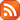Subscribe by RSS

# Operator Algebra Seminars

Fri., Apr. 5, 2019 10:30 a.m.

Location: Research and Innovation Centre (RI) 215

Speaker: Daniel Drimbe, University of Regina

Abstract:

A central theme in the classification of von Neumann algebras is the study of tensor product decompositions. A II$_1$ factor $M$ is called prime if it cannot be decomposed as a tensor product of II$_1$ factors.

In this talk we prove that any II$_1$ factor arising from a free strongly ergodic probability measure preserving action $\Gamma\car X$ of a product $\Gamma=\Gamma_1\times\dots\times\Gamma_n$ of icc hyperbolic, free product or wreath product groups is prime, unless $\Gamma\car X$ admits a non-trivial direct product decomposition. Using this we establish a unique prime factorization result for any such II$_1$ factor.